# sklearn.linear_model.BayesianRidge¶

class sklearn.linear_model.BayesianRidge(*, max_iter=None, tol=0.001, alpha_1=1e-06, alpha_2=1e-06, lambda_1=1e-06, lambda_2=1e-06, alpha_init=None, lambda_init=None, compute_score=False, fit_intercept=True, copy_X=True, verbose=False, n_iter='deprecated')[source]

Bayesian ridge regression.

Fit a Bayesian ridge model. See the Notes section for details on this implementation and the optimization of the regularization parameters lambda (precision of the weights) and alpha (precision of the noise).

Read more in the User Guide.

Parameters:
max_iterint, default=None

Maximum number of iterations over the complete dataset before stopping independently of any early stopping criterion. If None, it corresponds to max_iter=300.

Changed in version 1.3.

tolfloat, default=1e-3

Stop the algorithm if w has converged.

alpha_1float, default=1e-6

Hyper-parameter : shape parameter for the Gamma distribution prior over the alpha parameter.

alpha_2float, default=1e-6

Hyper-parameter : inverse scale parameter (rate parameter) for the Gamma distribution prior over the alpha parameter.

lambda_1float, default=1e-6

Hyper-parameter : shape parameter for the Gamma distribution prior over the lambda parameter.

lambda_2float, default=1e-6

Hyper-parameter : inverse scale parameter (rate parameter) for the Gamma distribution prior over the lambda parameter.

alpha_initfloat, default=None

Initial value for alpha (precision of the noise). If not set, alpha_init is 1/Var(y).

New in version 0.22.

lambda_initfloat, default=None

Initial value for lambda (precision of the weights). If not set, lambda_init is 1.

New in version 0.22.

compute_scorebool, default=False

If True, compute the log marginal likelihood at each iteration of the optimization.

fit_interceptbool, default=True

Whether to calculate the intercept for this model. The intercept is not treated as a probabilistic parameter and thus has no associated variance. If set to False, no intercept will be used in calculations (i.e. data is expected to be centered).

copy_Xbool, default=True

If True, X will be copied; else, it may be overwritten.

verbosebool, default=False

Verbose mode when fitting the model.

n_iterint

Maximum number of iterations. Should be greater than or equal to 1.

Deprecated since version 1.3: n_iter is deprecated in 1.3 and will be removed in 1.5. Use max_iter instead.

Attributes:
coef_array-like of shape (n_features,)

Coefficients of the regression model (mean of distribution)

intercept_float

Independent term in decision function. Set to 0.0 if fit_intercept = False.

alpha_float

Estimated precision of the noise.

lambda_float

Estimated precision of the weights.

sigma_array-like of shape (n_features, n_features)

Estimated variance-covariance matrix of the weights

scores_array-like of shape (n_iter_+1,)

If computed_score is True, value of the log marginal likelihood (to be maximized) at each iteration of the optimization. The array starts with the value of the log marginal likelihood obtained for the initial values of alpha and lambda and ends with the value obtained for the estimated alpha and lambda.

n_iter_int

The actual number of iterations to reach the stopping criterion.

X_offset_ndarray of shape (n_features,)

If fit_intercept=True, offset subtracted for centering data to a zero mean. Set to np.zeros(n_features) otherwise.

X_scale_ndarray of shape (n_features,)

Set to np.ones(n_features).

n_features_in_int

Number of features seen during fit.

New in version 0.24.

feature_names_in_ndarray of shape (n_features_in_,)

Names of features seen during fit. Defined only when X has feature names that are all strings.

New in version 1.0.

ARDRegression

Bayesian ARD regression.

Notes

There exist several strategies to perform Bayesian ridge regression. This implementation is based on the algorithm described in Appendix A of (Tipping, 2001) where updates of the regularization parameters are done as suggested in (MacKay, 1992). Note that according to A New View of Automatic Relevance Determination (Wipf and Nagarajan, 2008) these update rules do not guarantee that the marginal likelihood is increasing between two consecutive iterations of the optimization.

References

D. J. C. MacKay, Bayesian Interpolation, Computation and Neural Systems, Vol. 4, No. 3, 1992.

M. E. Tipping, Sparse Bayesian Learning and the Relevance Vector Machine, Journal of Machine Learning Research, Vol. 1, 2001.

Examples

>>> from sklearn import linear_model
>>> clf = linear_model.BayesianRidge()
>>> clf.fit([[0,0], [1, 1], [2, 2]], [0, 1, 2])
BayesianRidge()
>>> clf.predict([[1, 1]])
array([1.])


Methods

 fit(X, y[, sample_weight]) Fit the model. Get metadata routing of this object. get_params([deep]) Get parameters for this estimator. predict(X[, return_std]) Predict using the linear model. score(X, y[, sample_weight]) Return the coefficient of determination of the prediction. set_fit_request(*[, sample_weight]) Request metadata passed to the fit method. set_params(**params) Set the parameters of this estimator. set_predict_request(*[, return_std]) Request metadata passed to the predict method. set_score_request(*[, sample_weight]) Request metadata passed to the score method.
fit(X, y, sample_weight=None)[source]

Fit the model.

Parameters:
Xndarray of shape (n_samples, n_features)

Training data.

yndarray of shape (n_samples,)

Target values. Will be cast to X’s dtype if necessary.

sample_weightndarray of shape (n_samples,), default=None

Individual weights for each sample.

New in version 0.20: parameter sample_weight support to BayesianRidge.

Returns:
selfobject

Returns the instance itself.

Get metadata routing of this object.

Please check User Guide on how the routing mechanism works.

Returns:

A MetadataRequest encapsulating routing information.

get_params(deep=True)[source]

Get parameters for this estimator.

Parameters:
deepbool, default=True

If True, will return the parameters for this estimator and contained subobjects that are estimators.

Returns:
paramsdict

Parameter names mapped to their values.

predict(X, return_std=False)[source]

Predict using the linear model.

In addition to the mean of the predictive distribution, also its standard deviation can be returned.

Parameters:
X{array-like, sparse matrix} of shape (n_samples, n_features)

Samples.

return_stdbool, default=False

Whether to return the standard deviation of posterior prediction.

Returns:
y_meanarray-like of shape (n_samples,)

Mean of predictive distribution of query points.

y_stdarray-like of shape (n_samples,)

Standard deviation of predictive distribution of query points.

score(X, y, sample_weight=None)[source]

Return the coefficient of determination of the prediction.

The coefficient of determination $$R^2$$ is defined as $$(1 - \frac{u}{v})$$, where $$u$$ is the residual sum of squares ((y_true - y_pred)** 2).sum() and $$v$$ is the total sum of squares ((y_true - y_true.mean()) ** 2).sum(). The best possible score is 1.0 and it can be negative (because the model can be arbitrarily worse). A constant model that always predicts the expected value of y, disregarding the input features, would get a $$R^2$$ score of 0.0.

Parameters:
Xarray-like of shape (n_samples, n_features)

Test samples. For some estimators this may be a precomputed kernel matrix or a list of generic objects instead with shape (n_samples, n_samples_fitted), where n_samples_fitted is the number of samples used in the fitting for the estimator.

yarray-like of shape (n_samples,) or (n_samples, n_outputs)

True values for X.

sample_weightarray-like of shape (n_samples,), default=None

Sample weights.

Returns:
scorefloat

$$R^2$$ of self.predict(X) w.r.t. y.

Notes

The $$R^2$$ score used when calling score on a regressor uses multioutput='uniform_average' from version 0.23 to keep consistent with default value of r2_score. This influences the score method of all the multioutput regressors (except for MultiOutputRegressor).

set_fit_request(*, sample_weight: Union[bool, None, str] = '$UNCHANGED$') [source]

Request metadata passed to the fit method.

Note that this method is only relevant if enable_metadata_routing=True (see sklearn.set_config). Please see User Guide on how the routing mechanism works.

The options for each parameter are:

• True: metadata is requested, and passed to fit if provided. The request is ignored if metadata is not provided.

• False: metadata is not requested and the meta-estimator will not pass it to fit.

• None: metadata is not requested, and the meta-estimator will raise an error if the user provides it.

• str: metadata should be passed to the meta-estimator with this given alias instead of the original name.

The default (sklearn.utils.metadata_routing.UNCHANGED) retains the existing request. This allows you to change the request for some parameters and not others.

New in version 1.3.

Note

This method is only relevant if this estimator is used as a sub-estimator of a meta-estimator, e.g. used inside a pipeline.Pipeline. Otherwise it has no effect.

Parameters:
sample_weightstr, True, False, or None, default=sklearn.utils.metadata_routing.UNCHANGED

Metadata routing for sample_weight parameter in fit.

Returns:
selfobject

The updated object.

set_params(**params)[source]

Set the parameters of this estimator.

The method works on simple estimators as well as on nested objects (such as Pipeline). The latter have parameters of the form <component>__<parameter> so that it’s possible to update each component of a nested object.

Parameters:
**paramsdict

Estimator parameters.

Returns:
selfestimator instance

Estimator instance.

set_predict_request(*, return_std: Union[bool, None, str] = '$UNCHANGED$') [source]

Request metadata passed to the predict method.

Note that this method is only relevant if enable_metadata_routing=True (see sklearn.set_config). Please see User Guide on how the routing mechanism works.

The options for each parameter are:

• True: metadata is requested, and passed to predict if provided. The request is ignored if metadata is not provided.

• False: metadata is not requested and the meta-estimator will not pass it to predict.

• None: metadata is not requested, and the meta-estimator will raise an error if the user provides it.

• str: metadata should be passed to the meta-estimator with this given alias instead of the original name.

The default (sklearn.utils.metadata_routing.UNCHANGED) retains the existing request. This allows you to change the request for some parameters and not others.

New in version 1.3.

Note

This method is only relevant if this estimator is used as a sub-estimator of a meta-estimator, e.g. used inside a pipeline.Pipeline. Otherwise it has no effect.

Parameters:
return_stdstr, True, False, or None, default=sklearn.utils.metadata_routing.UNCHANGED

Metadata routing for return_std parameter in predict.

Returns:
selfobject

The updated object.

set_score_request(*, sample_weight: Union[bool, None, str] = '$UNCHANGED$') [source]

Request metadata passed to the score method.

Note that this method is only relevant if enable_metadata_routing=True (see sklearn.set_config). Please see User Guide on how the routing mechanism works.

The options for each parameter are:

• True: metadata is requested, and passed to score if provided. The request is ignored if metadata is not provided.

• False: metadata is not requested and the meta-estimator will not pass it to score.

• None: metadata is not requested, and the meta-estimator will raise an error if the user provides it.

• str: metadata should be passed to the meta-estimator with this given alias instead of the original name.

The default (sklearn.utils.metadata_routing.UNCHANGED) retains the existing request. This allows you to change the request for some parameters and not others.

New in version 1.3.

Note

This method is only relevant if this estimator is used as a sub-estimator of a meta-estimator, e.g. used inside a pipeline.Pipeline. Otherwise it has no effect.

Parameters:
sample_weightstr, True, False, or None, default=sklearn.utils.metadata_routing.UNCHANGED

Metadata routing for sample_weight parameter in score.

Returns:
selfobject

The updated object.

## Examples using sklearn.linear_model.BayesianRidge¶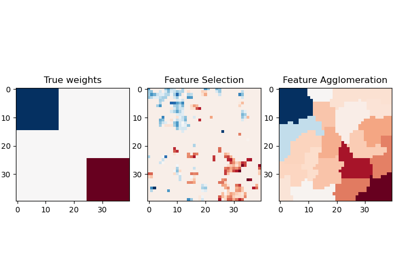Feature agglomeration vs. univariate selection

Feature agglomeration vs. univariate selection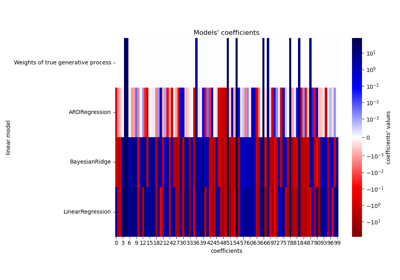Comparing Linear Bayesian Regressors

Comparing Linear Bayesian Regressors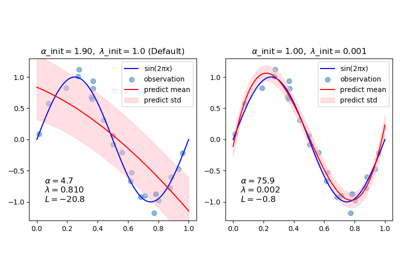Curve Fitting with Bayesian Ridge Regression

Curve Fitting with Bayesian Ridge Regression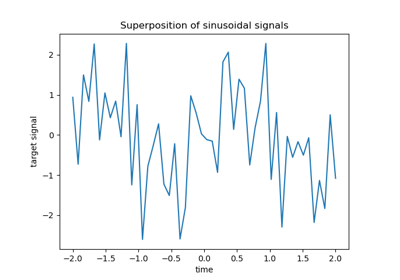L1-based models for Sparse Signals

L1-based models for Sparse Signals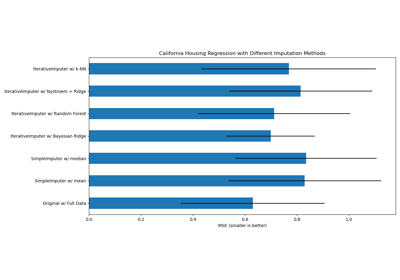Imputing missing values with variants of IterativeImputer

Imputing missing values with variants of IterativeImputer